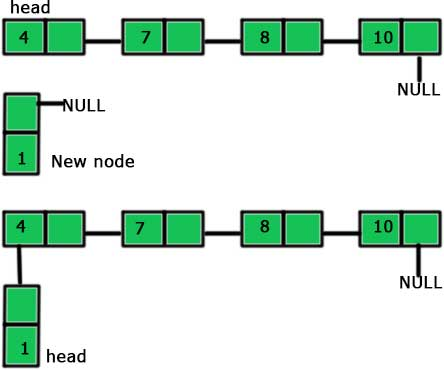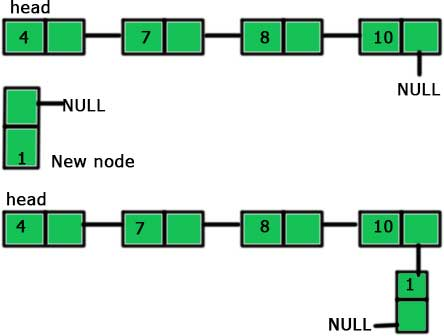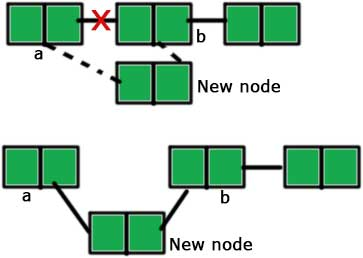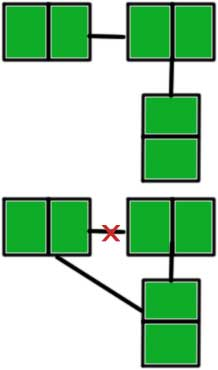# Inserting a new node to a linked list in C++

May 30, 2017 260019

### Previous:

Make sure that you are familiar with the concepts explained in the post(s) mentioned above before proceeding further.

We will proceed further by taking the linked list we made in the previous post.

#include <iostream>

using namespace std;

struct node
{
int data;
node *next;
};

{
private:
public:
{
tail = NULL;
}

{
node *tmp = new node;
tmp->data = n;
tmp->next = NULL;

{
tail = tmp;
}
else
{
tail->next = tmp;
tail = tail->next;
}
}

{
}

{
{
cout << "NULL" << endl;
}
else
{
}
}

static void concatenate(node *a,node *b)
{
if( a != NULL && b!= NULL )
{
if (a->next == NULL)
a->next = b;
else
concatenate(a->next,b);
}
else
{
cout << "Either a or b is NULL\n";
}
}
};

int main()
{
return 0;
}


There are three different possibilities for inserting a node into a linked list. These three possibilities are:

1. Insertion at the beginning of the list.
2. Insertion at the end of the list
3. Inserting a new node anywhere in between the list

In the first case, we make a new node and points its next to the head of the existing list and then change the head to the newly added node. It is similar to picture given below.So, the steps to be followed are as follows:

1. Make a new node
2. Point the ‘next’ of the new node to the ‘head’ of the linked list.
3. Mark new node as ‘head’.

Thus, the code representing the above steps is:

void front(int n)
{
node *tmp = new node;
tmp -> data = n;
}


The code is very simple to understand. We just made a new node first – node *tmp = new node;

tmp->next=head – In this line, we have followed the second step which is to point the ‘next’ of the new node to the head of the linked list.

And in the last line, we are making the new node ‘head’ as per the third step – head = tmp;

The second case is the simplest one. We just add a new node at the end of the existing list. It is shown in the picture given below:So, the steps to add the end if a linked list are:

1. Make a new node
2. Point the last node of the linked list to the new node

We have already dealt with this in the first post with the ‘add_node’ function. I am just mentioning the ‘add_node’ function here again.

void add_node(int n)
{
node *tmp = new node;
tmp->data = n;
tmp->next = NULL;

{
tail = tmp;
}
else
{
tail->next = tmp;
tail = tail->next;
}
}


You can read the explanation of the above code in the first post of linked list.

The third and the last case is a little bit complicated. To insert a node in between a linked list, we need to first break the existing link and then create two new links. It will be clear from the picture given below.The steps for inserting a node after node ‘a’ (as shown in the picture) are:

1. Make a new node
2. Point the ‘next’ of the new node to the node ‘b’ (the node after which we have to insert the new node). Till now, two nodes are pointing the same node ‘b’, the node ‘a’ and the new node.3. Point the ‘next’ of ‘a’ to the new node.

The code for the above steps is:

    void after(node *a, int value)
{
node* p = new node;
p->data = value;
/*
_______        _______        _______
|   1   |____\ |   3   |____\ |   5   |____\ NULL
|_______|    / |_______|    / |_______|    /
and new node's value is 10
then the next line will do something like
_______        _______        _______
|   1   |____\ |   3   |____\ |   5   |____\ NULL
|_______|    / |_______|    / |_______|    /
/ \
|
|
___|___
|   10  |
|_______|
*/
p->next = a->next;
a->next = p;
/*
now the linked list will look like:
_______       _______        _______        _______
|   1   |____\|   10  |____\ |   3   |____\ |   5   |____\ NULL
|_______|    /|_______|    / |_______|    / |_______|    /
*/
}


node* p = new node; – We are creating a new node.

p->next = a->next – We are making the ‘next’ of the new node to point to the node after which insertion is to be made. See the comments for better understanding.

a->next = p – We are pointing the ‘next’ of ‘a’ to the new node.

The entire code is:

#include <iostream>

using namespace std;

struct node
{
int data;
node *next;
};

{
private:
public:
{
tail = NULL;
}

{
node *tmp = new node;
tmp->data = n;
tmp->next = NULL;

{
tail = tmp;
}
else
{
tail->next = tmp;
tail = tail->next;
}
}

{
}

{
{
cout << "NULL" << endl;
}
else
{
}
}

static void concatenate(node *a,node *b)
{
if( a != NULL && b!= NULL )
{
if (a->next == NULL)
a->next = b;
else
concatenate(a->next,b);
}
else
{
cout << "Either a or b is NULL\n";
}
}

void front(int n)
{
node *tmp = new node;
tmp -> data = n;
}

void after(node *a, int value)
{
node* p = new node;
p->data = value;
p->next = a->next;
a->next = p;
}
};

int main()
{
a.front(3);